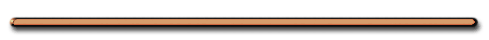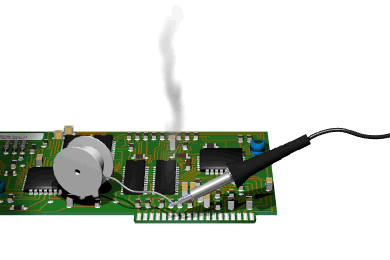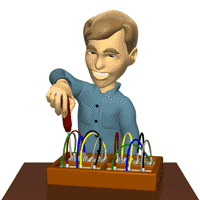D) Conditions for passing current

[ Flash ]

Voltage, Resistance and Current

1) complete circuit2) potential difference (voltage) supplied by some source

ex. Battery, generator

3) conductor (wires)I. Important Circuit Terms

A) Current (I) - measure of charge flowScientific Advisors: Dr. Saul Dushman; Dr. Roman Smoluchowski; Dr. David Harker

Production Company: Wolff (Raphael G.) Studios, Inc. Audio/Visual: sound, color

Full Size Current Video

 I = q/t

q = charge (C)

t = time (sec)

= (C)/(sec) UNIT

OR Amperes

 Ex) If 10. coulombs of charge are transferred through an electric circuit in 5.0 seconds, then the current in the circuit is

Ex) If 10. coulombs of charge are transferred through an electric circuit in 5.0 seconds, then the current in the circuit is

I = 10. C/5.0 sec

I = 2.0 Amps

1 Coulomb = 6.25 x 1018 electrons

(A bundle of Electrons)

p. 1 Reference

 I = (#e-)(1.6 x 10-19 C) t

 Ex) A wire carries a current of 2.0 amperes. How many electrons pass a given point in this wire in 1.0 sec?

Ex) A wire carries a current of 2.0 amperes. How many electrons pass a given point in this wire in 1.0 sec?

 I = (#e-)(1.6 x 10-19C) t

 2.0 A = (#e-)(1.6 x 10-19 C) 1.0 sec

Cross Multiply And Divide

 2.0 A = (#e-) (1.6 x 10-19  C)

#e- = 1.3 x 1019

(2 sig. figs.)

AP Physics

Giancoli - p. 515) 8

V = IR

9.0 V = I[1.6 ohm]

I = 5.6 Amps

I = q/t

q  = It

q = 5.6A(60 sec)

= 330 C

divide by  1.6 x 10-19C

= 2.1 x 1021 elect.